Part of the "Understanding monoids" series (link)

# Monoids in practice

Monoids without tears - Part 2

In the previous post, we looked at the definition of a monoid. In this post, we’ll see how to implement some monoids.

First, let’s revisit the definition:

• You start with a bunch of things, and some way of combining them two at a time.
• Rule 1 (Closure): The result of combining two things is always another one of the things.
• Rule 2 (Associativity): When combining more than two things, which pairwise combination you do first doesn’t matter.
• Rule 3 (Identity element): There is a special thing called “zero” such that when you combine any thing with “zero” you get the original thing back.

For example, if strings are the things, and string concatenation is the operation, then we have a monoid. Here’s some code that demonstrates this:

``````let s1 = "hello"
let s2 = " world!"

// closure
let sum = s1 + s2  // sum is a string

// associativity
let s3 = "x"
let s4a = (s1+s2) + s3
let s4b = s1 + (s2+s3)
assert (s4a = s4b)

// an empty string is the identity
assert (s1 + "" = s1)
assert ("" + s1 = s1)
``````

But now let’s try to apply this to a more complicated object.

Say that we have an `OrderLine`, a little structure that represents a line in a sales order, say.

``````type OrderLine = {
ProductCode: string
Qty: int
Total: float
}
``````

And then perhaps we might want to find the total for an order, that is, we want to sum the `Total` field for a list of lines.

The standard imperative approach would be to create a local `total` variable, and then loop through the lines, summing as we go, like this:

``````let calculateOrderTotal lines =
let mutable total = 0.0
for line in lines do
total <- total + line.Total
total
``````

Let’s try it:

``````module OrdersUsingImperativeLoop =

type OrderLine = {
ProductCode: string
Qty: int
Total: float
}

let calculateOrderTotal lines =
let mutable total = 0.0
for line in lines do
total <- total + line.Total
total

let orderLines = [
{ProductCode="AAA"; Qty=2; Total=19.98}
{ProductCode="BBB"; Qty=1; Total=1.99}
{ProductCode="CCC"; Qty=3; Total=3.99}
]

orderLines
|> calculateOrderTotal
|> printfn "Total is %g"
``````

But of course, being an experienced functional programmer, you would sneer at this, and use `fold` in `calculateOrderTotal` instead, like this:

``````module OrdersUsingFold =

type OrderLine = {
ProductCode: string
Qty: int
Total: float
}

let calculateOrderTotal lines =
let accumulateTotal total line =
total + line.Total
lines
|> List.fold accumulateTotal 0.0

let orderLines = [
{ProductCode="AAA"; Qty=2; Total=19.98}
{ProductCode="BBB"; Qty=1; Total=1.99}
{ProductCode="CCC"; Qty=3; Total=3.99}
]

orderLines
|> calculateOrderTotal
|> printfn "Total is %g"
``````

So far, so good. Now let’s look at a solution using a monoid approach.

For a monoid, we need to define some sort of addition or combination operation. How about something like this?

``````let addLine orderLine1 orderLine2 =
orderLine1.Total + orderLine2.Total
``````

But this is no good, because we forgot a key aspect of monoids. The addition must return a value of the same type!

If we look at the signature for the `addLine` function…

``````addLine : OrderLine -> OrderLine -> float
``````

…we can see that the return type is `float` not `OrderLine`.

What we need to do is return a whole other `OrderLine`. Here’s a correct implementation:

``````let addLine orderLine1 orderLine2 =
{
ProductCode = "TOTAL"
Qty = orderLine1.Qty + orderLine2.Qty
Total = orderLine1.Total + orderLine2.Total
}
``````

Now the signature is correct: `addLine : OrderLine -> OrderLine -> OrderLine`.

Note that because we have to return the entire structure we have to specify something for the `ProductCode` and `Qty` as well, not just the total. The `Qty` is easy, we can just do a sum. For the `ProductCode`, I decided to use the string “TOTAL”, because we don’t have a real product code we can use.

Let’s give this a little test:

``````// utility method to print an OrderLine
let printLine {ProductCode=p; Qty=q;Total=t} =
printfn "%-10s %5i %6g" p q t

let orderLine1 = {ProductCode="AAA"; Qty=2; Total=19.98}
let orderLine2 = {ProductCode="BBB"; Qty=1; Total=1.99}

//add two lines to make a third
let orderLine3 = addLine orderLine1 orderLine2
orderLine3 |> printLine // and print it
``````

We should get this result:

``````TOTAL          3  21.97
``````

NOTE: For more on the printf formatting options used, see the post on printf here.

Now let’s apply this to a list using `reduce`:

``````let orderLines = [
{ProductCode="AAA"; Qty=2; Total=19.98}
{ProductCode="BBB"; Qty=1; Total=1.99}
{ProductCode="CCC"; Qty=3; Total=3.99}
]

orderLines
|> printLine
``````

With the result:

``````TOTAL          6  25.96
``````

At first, this might seem like extra work, and just to add up a total. But note that we now have more information than just the total; we also have the sum of the qtys as well.

For example, we can easily reuse the `printLine` function to make a simple receipt printing function that includes the total, like this:

``````let printReceipt lines =
lines
|> List.iter printLine

printfn "-----------------------"

lines
|> printLine

orderLines
|> printReceipt
``````

Which gives an output like this:

``````AAA            2  19.98
BBB            1   1.99
CCC            3   3.99
-----------------------
TOTAL          6  25.96
``````

More importantly, we can now use the incremental nature of monoids to keep a running subtotal that we update every time a new line is added.

Here’s an example:

``````let subtotal = orderLines |> List.reduce addLine
let newLine = {ProductCode="DDD"; Qty=1; Total=29.98}
let newSubtotal = subtotal |> addLine newLine
newSubtotal |> printLine
``````

We could even define a custom operator such as `++` so that we can add lines together naturally as it they were numbers:

``````let (++) a b = addLine a b  // custom operator

let newSubtotal = subtotal ++ newLine
``````

You can see that using the monoid pattern opens up a whole new way of thinking. You can apply this “add” approach to almost any kind of object.

For example, what would a product “plus” a product look like? Or a customer “plus” a customer? Let your imagination run wild!

### Are we there yet?

You might have noticed that we not quite done yet. There is a third requirement for a monoid that we haven’t discussed yet – the zero or identity element.

In this case, the requirement means that we need some kind of `OrderLine` such that adding it to another order line would leave the original untouched. Do we have such a thing?

Right now, no, because the addition operation always changes the product code to “TOTAL”. What we have right now is in fact a semigroup, not a monoid.

As you can see, a semigroup is perfectly usable. But a problem would arise if we had an empty list of lines and we wanted to total them. What should the result be?

One workaround would be to change the `addLine` function to ignore empty product codes. And then we could use an order line with an empty code as the zero element.

Here’s what I mean:

``````let addLine orderLine1 orderLine2 =
match orderLine1.ProductCode, orderLine2.ProductCode with
// is one of them zero? If so, return the other one
| "", _ -> orderLine2
| _, "" -> orderLine1
// anything else is as before
| _ ->
{
ProductCode = "TOTAL"
Qty = orderLine1.Qty + orderLine2.Qty
Total = orderLine1.Total + orderLine2.Total
}

let zero = {ProductCode=""; Qty=0; Total=0.0}
let orderLine1 = {ProductCode="AAA"; Qty=2; Total=19.98}
``````

We can then test that identity works as expected:

``````assert (orderLine1 = addLine orderLine1 zero)
assert (orderLine1 = addLine zero orderLine1)
``````

This does seem a bit hacky, so I wouldn’t recommend this technique in general. There’s another way to get an identity that we’ll be discussing later.

## Introducing a special total type

In the example above, the `OrderLine` type was very simple and it was easy to overload the fields for the total.

But what would happen if the `OrderLine` type was more complicated? For example, if it had a `Price` field as well, like this:

``````type OrderLine = {
ProductCode: string
Qty: int
Price: float
Total: float
}
``````

Now we have introduced a complication. What should we set the `Price` to when we combine two lines? The average price? No price?

``````let addLine orderLine1 orderLine2 =
{
ProductCode = "TOTAL"
Qty = orderLine1.Qty + orderLine2.Qty
Price = 0 // or use average price?
Total = orderLine1.Total + orderLine2.Total
}
``````

Neither seems very satisfactory.

The fact that we don’t know what to do probably means that our design is wrong.

Really, we only need a subset of the data for the total, not all of it. How can we represent this?

With a discriminated union of course! One case can be used for product lines, and the other case can be used for totals only.

Here’s what I mean:

``````type ProductLine = {
ProductCode: string
Qty: int
Price: float
LineTotal: float
}

type TotalLine = {
Qty: int
OrderTotal: float
}

type OrderLine =
| Product of ProductLine
| Total of TotalLine
``````

This design is much nicer. We now have a special structure just for totals and we don’t have to use contortions to make the excess data fit. We can even remove the dummy “TOTAL” product code.

Note that I named the “total” field differently in each record. Having unique field names like this means that you don’t have to always specify the type explicitly.

Unfortunately, the addition logic is more complicated now, as we have to handle every combination of cases:

``````let addLine orderLine1 orderLine2 =
let totalLine =
match orderLine1,orderLine2 with
| Product p1, Product p2 ->
{Qty = p1.Qty + p2.Qty;
OrderTotal = p1.LineTotal + p2.LineTotal}
| Product p, Total t ->
{Qty = p.Qty + t.Qty;
OrderTotal = p.LineTotal + t.OrderTotal}
| Total t, Product p ->
{Qty = p.Qty + t.Qty;
OrderTotal = p.LineTotal + t.OrderTotal}
| Total t1, Total t2 ->
{Qty = t1.Qty + t2.Qty;
OrderTotal = t1.OrderTotal + t2.OrderTotal}
Total totalLine // wrap totalLine to make OrderLine
``````

Note that we cannot just return the `TotalLine` value. We have to wrap in the `Total` case to make a proper `OrderLine`. If we didn’t do that, then our `addLine` would have the signature `OrderLine -> OrderLine -> TotalLine`, which is not correct. We have to have the signature `OrderLine -> OrderLine -> OrderLine` – nothing else will do!

Now that we have two cases, we need to handle both of them in the `printLine` function:

``````let printLine =  function
| Product {ProductCode=p; Qty=q; Price=pr; LineTotal=t} ->
printfn "%-10s %5i @%4g each %6g" p q pr t
| Total {Qty=q; OrderTotal=t} ->
printfn "%-10s %5i            %6g" "TOTAL" q t
``````

But once we have done this, we can now use addition just as before:

``````let orderLine1 = Product {ProductCode="AAA"; Qty=2; Price=9.99; LineTotal=19.98}
let orderLine2 = Product {ProductCode="BBB"; Qty=1; Price=1.99; LineTotal=1.99}
let orderLine3 = addLine orderLine1 orderLine2

orderLine1 |> printLine
orderLine2 |> printLine
orderLine3 |> printLine
``````

### Identity again

Again, we haven’t dealt with the identity requirement. We could try using the same trick as before, with a blank product code, but that only works with the `Product` case.

To get a proper identity, we really need to introduce a third case, `EmptyOrder` say, to the union type:

``````type ProductLine = {
ProductCode: string
Qty: int
Price: float
LineTotal: float
}

type TotalLine = {
Qty: int
OrderTotal: float
}

type OrderLine =
| Product of ProductLine
| Total of TotalLine
| EmptyOrder
``````

With this extra case available, we rewrite the `addLine` function to handle it:

``````let addLine orderLine1 orderLine2 =
match orderLine1,orderLine2 with
// is one of them zero? If so, return the other one
| EmptyOrder, _ -> orderLine2
| _, EmptyOrder -> orderLine1
// otherwise as before
| Product p1, Product p2 ->
Total { Qty = p1.Qty + p2.Qty;
OrderTotal = p1.LineTotal + p2.LineTotal}
| Product p, Total t ->
Total {Qty = p.Qty + t.Qty;
OrderTotal = p.LineTotal + t.OrderTotal}
| Total t, Product p ->
Total {Qty = p.Qty + t.Qty;
OrderTotal = p.LineTotal + t.OrderTotal}
| Total t1, Total t2 ->
Total {Qty = t1.Qty + t2.Qty;
OrderTotal = t1.OrderTotal + t2.OrderTotal}
``````

And now we can test it:

``````let zero = EmptyOrder

// test identity
let productLine = Product {ProductCode="AAA"; Qty=2; Price=9.99; LineTotal=19.98}
assert (productLine = addLine productLine zero)
assert (productLine = addLine zero productLine)

let totalLine = Total {Qty=2; OrderTotal=19.98}
assert (totalLine = addLine totalLine zero)
assert (totalLine = addLine zero totalLine)
``````

## Using the built in List.sum function

It turns out that the `List.sum` function knows about monoids! If you tell it what the addition operation is, and what the zero is, then you can use `List.sum` directly rather than `List.fold`.

The way you do this is by attaching two static members, `+` and `Zero` to your type, like this:

``````type OrderLine with
static member (+) (x,y) = addLine x y
static member Zero = EmptyOrder   // a property
``````

Once this has been done, you can use `List.sum` and it will work as expected.

``````let lines1 = [productLine]
// using fold with explicit op and zero
lines1 |> List.fold addLine zero |> printfn "%A"
// using sum with implicit op and zero
lines1 |> List.sum |> printfn "%A"

let emptyList: OrderLine list = []
// using fold with explicit op and zero
emptyList |> List.fold addLine zero |> printfn "%A"
// using sum with implicit op and zero
emptyList |> List.sum |> printfn "%A"
``````

Note that for this to work you mustn’t already have a method or case called `Zero`. If I had used the name `Zero` instead of `EmptyOrder` for the third case it would not have worked.

Although this is a neat trick, in practice I don’t think it is a good idea unless you are defining a proper math-related type such as `ComplexNumber` or `Vector`. It’s a bit too clever and non-obvious for my taste.

If you do want to use this trick, your `Zero` member cannot be an extension method – it must be defined with the type.

For example, in the code below, I’m trying to define the empty string as the “zero” for strings.

`List.fold` works because `String.Zero` is visible as an extension method in this code right here, but `List.sum` fails because the extension method is not visible to it.

``````module StringMonoid =

// define extension method
type System.String with
static member Zero = ""

// OK.
["a";"b";"c"]
|> List.reduce (+)
|> printfn "Using reduce: %s"

// OK. String.Zero is visible as an extension method
["a";"b";"c"]
|> List.fold (+) System.String.Zero
|> printfn "Using fold: %s"

// Error. String.Zero is NOT visible to List.sum
["a";"b";"c"]
|> List.sum
|> printfn "Using sum: %s"
``````

## Mapping to a different structure

Having two different cases in a union might be acceptable in the order line case, but in many real world cases, that approach is too complicated or confusing.

Consider a customer record like this:

``````open System

type Customer = {
Name:string // and many more string fields!
LastActive:DateTime
TotalSpend:float }
``````

How would we “add” two of these customers?

A helpful tip is to realize that aggregation really only works for numeric and similar types. Strings can’t really be aggregated easily.

So rather than trying to aggregate `Customer`, let’s define a separate class `CustomerStats` that contains all the aggregatable information:

``````// create a type to track customer statistics
type CustomerStats = {
// number of customers contributing to these stats
Count:int
// total number of days since last activity
TotalInactiveDays:int
// total amount of money spent
TotalSpend:float }
``````

All the fields in `CustomerStats` are numeric, so it is obvious how we can add two stats together:

``````let add stat1 stat2 = {
Count = stat1.Count + stat2.Count;
TotalInactiveDays = stat1.TotalInactiveDays + stat2.TotalInactiveDays
TotalSpend = stat1.TotalSpend + stat2.TotalSpend
}

// define an infix version as well
let (++) a b = add a b
``````

As always, the inputs and output of the `add` function must be the same type. We must have `CustomerStats -> CustomerStats -> CustomerStats`, not `Customer -> Customer -> CustomerStats` or any other variant.

Ok, so far so good.

Now let’s say we have a collection of customers, and we want to get the aggregated stats for them, how should we do this?

We can’t add the customers directly, so what we need to do is first convert each customer to a `CustomerStats`, and then add the stats up using the monoid operation.

Here’s an example:

``````// convert a customer to a stat
let toStats cust =
let inactiveDays= DateTime.Now.Subtract(cust.LastActive).Days;
{Count=1; TotalInactiveDays=inactiveDays; TotalSpend=cust.TotalSpend}

// create a list of customers
let c1 = {Name="Alice"; LastActive=DateTime(2005,1,1); TotalSpend=100.0}
let c2 = {Name="Bob"; LastActive=DateTime(2010,2,2); TotalSpend=45.0}
let c3 = {Name="Charlie"; LastActive=DateTime(2011,3,3); TotalSpend=42.0}
let customers = [c1;c2;c3]

// aggregate the stats
customers
|> List.map toStats
|> printfn "result = %A"
``````

The first thing to note is that the `toStats` creates statistics for just one customer. We set the count to just 1. It might seem a bit strange, but it does make sense, because if there was just one customer in the list, that’s what the aggregate stats would be.

The second thing to note is how the final aggregation is done. First we use `map` to convert the source type to a type that is a monoid, and then we use `reduce` to aggregate all the stats.

Hmmm…. `map` followed by `reduce`. Does that sound familiar to you?

Yes indeed, Google’s famous MapReduce algorithm was inspired by this concept (although the details are somewhat different).

Before we move on, here are some simple exercises for you to check your understanding.

• What is the “zero” for `CustomerStats`? Test your code by using `List.fold` on an empty list.
• Write a simple `OrderStats` class and use it to aggregate the `OrderLine` type that we introduced at the beginning of this post.

## Monoid Homomorphisms

We’ve now got all the tools we need to understand something called a monoid homomorphism.

I know what you’re thinking… Ugh! Not just one, but two strange math words at once!

But I hope that the word “monoid” is not so intimidating now. And “homomorphism” is another math word that is simpler than it sounds. It’s just greek for “same shape” and it describes a mapping or function that keeps the “shape” the same.

What does that mean in practice?

Well, we have seen that all monoids have a certain common structure. That is, even though the underlying objects can be quite different (integers, strings, lists, `CustomerStats`, etc.) the “monoidness” of them is the same. As George W. Bush once said, once you’ve seen one monoid, you’ve seen them all.

So a monoid homomorphism is a transformation that preserves an essential “monoidness”, even if the “before” and “after” objects are quite different.

In this section, we’ll look at a simple monoid homomorphism. It’s the “hello world”, the “fibonacci series”, of monoid homomorphisms – word counting.

### Documents as a monoid

Let’s say we have a type which represents text blocks, something like this:

``````type Text = Text of string
``````

And of course we can add two smaller text blocks to make a larger text block:

``````let addText (Text s1) (Text s2) =
Text (s1 + s2)
``````

Here’s an example of how adding works:

``````let t1 = Text "Hello"
let t2 = Text " World"
let t3 = addText t1 t2
``````

Since you are now a expert, you will quickly recognize this as a monoid, with the zero obviously being `Text ""`.

Now let’s say we are writing a book (such as this one) and we want a word count to show how much we have written.

Here’s a very crude implementation, plus a test:

``````let wordCount (Text s) =
s.Split(' ').Length

// test
Text "Hello world"
|> wordCount
|> printfn "The word count is %i"
``````

So we are writing away, and now we have produced three pages of text. How do we calculate the word count for the complete document?

Well, one way is to add the separate pages together to make a complete text block, and then apply the `wordCount` function to that text block. Here’s a diagram: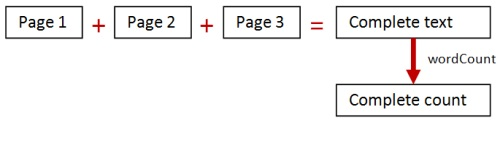But every time we finish a new page, we have to add all the text together and do the word count all over again.

No doubt you can see that there is a better way of doing this. Instead of adding all the text together and then counting, get the word count for each page separately, and then add these counts up, like this: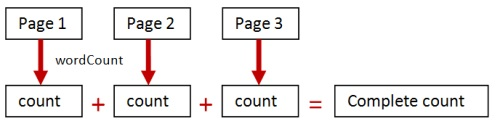The second approach relies on the fact that integers (the counts) are themselves a monoid, and you can add them up to get the desired result.

So the `wordCount` function has transformed an aggregation over “pages” into an aggregation over “counts”.

The big question now: is `wordCount` a monoid homomorphism?

Well, pages (text) and counts (integers) are both monoids, so it certainly transforms one monoid into another.

But the more subtle condition is: does it preserve the “shape”? That is, does the adding of the counts give the same answer as the adding of the pages?

In this case, the answer is yes. So `wordCount` is a monoid homomorphism!

You might think that this is obvious, and that all mappings like this must be monoid homomorphisms, but we’ll see an example later where this is not true.

### The benefits of chunkability

The advantage of the monoid homomorphism approach is that it is “chunkable”.

Each map and word count is independent of the others, so we can do them separately and then add up the answers afterwards. For many algorithms, working on small chunks of data is much more efficient than working on large chunks, so if we can, we should exploit this whenever possible.

As a direct consequence of this chunkability, we get some of the benefits that we touched on in the previous post.

First, it is incremental. That is, as we add text to the last page, we don’t have to recalculate the word counts for all the previous pages, which might save some time.

Second, it is parallelizable. The work for each chunk can be done independently, on different cores or machines. Note that in practice, parallelism is much overrated. The chunkability into small pieces has a much greater effect on performance than parallelism itself.

### Comparing word count implementations

We’re now ready to create some code that will demonstrate these two different techniques.

Let’s start with the basic definitions from above, except that I will change the word count to use regular expressions instead of `split`.

``````module WordCountTest =
open System

type Text = Text of string

let addText (Text s1) (Text s2) =
Text (s1 + s2)

let wordCount (Text s) =
System.Text.RegularExpressions.Regex.Matches(s,@"\S+").Count
``````

Next, we’ll create a page with 1000 words in it, and a document with 1000 pages.

``````module WordCountTest =

// code as above

let page() =
List.replicate 1000 "hello "
|> List.reduce (+)
|> Text

let document() =
page() |> List.replicate 1000
``````

We’ll want to time the code to see if there is any difference between the implementations. Here’s a little helper function.

``````module WordCountTest =

// code as above

let time f msg =
let stopwatch = Diagnostics.Stopwatch()
stopwatch.Start()
f()
stopwatch.Stop()
printfn "Time taken for %s was %ims" msg stopwatch.ElapsedMilliseconds
``````

Ok, let’s implement the first approach. We’ll add all the pages together using `addText` and then do a word count on the entire million word document.

``````module WordCountTest =

// code as above

document()
|> wordCount
|> printfn "The word count is %i"

``````

For the second approach, we’ll do `wordCount` on each page first, and then add all the results together (using `reduce` of course).

``````module WordCountTest =

// code as above

let wordCountViaMap() =
document()
|> List.map wordCount
|> List.reduce (+)
|> printfn "The word count is %i"

time wordCountViaMap "map then reduce"
``````

Note that we have only changed two lines of code!

In `wordCountViaAddText` we had:

``````|> List.reduce addText
|> wordCount
``````

And in `wordCountViaMap` we have basically swapped these lines. We now do `wordCount` first and then `reduce` afterwards, like this:

``````|> List.map wordCount
|> List.reduce (+)
``````

Finally, let’s see what difference parallelism makes. We’ll use the built-in `Array.Parallel.map` instead of `List.map`, which means we’ll need to convert the list into an array first.

``````module WordCountTest =

// code as above

document()
|> List.toArray
|> Array.Parallel.map wordCount
|> Array.reduce (+)
|> printfn "The word count is %i"

time wordCountViaParallelAddCounts "parallel map then reduce"
``````

I hope that you are following along with the implementations, and that you understand what is going on.

### Analyzing the results

Here are the results for the different implementations running on my 4 core machine:

``````Time taken for reduce then count was 7955ms
Time taken for map then reduce was 698ms
Time taken for parallel map then reduce was 603ms
``````

We must recognize that these are crude results, not a proper performance profile. But even so, it is very obvious that the map/reduce version is about 10 times faster that the `ViaAddText` version.

This is the key to why monoid homomorphisms are important – they enable a “divide and conquer” strategy that is both powerful and easy to implement.

Yes, you could argue that the algorithms used are very inefficient. String concat is a terrible way to accumulate large text blocks, and there are much better ways of doing word counts. But even with these caveats, the fundamental point is still valid: by swapping two lines of code, we got a huge performance increase.

And with a little bit of hashing and caching, we would also get the benefits of incremental aggregation – only recalculating the minimum needed as pages change.

Note that the parallel map didn’t make that much difference in this case, even though it did use all four cores. Yes, we did add some minor expense with `toArray` but even in the best case, you might only get a small speed up on a multicore machine. To reiterate, what really made the most difference was the divide and conquer strategy inherent in the map/reduce approach.

## A non-monoid homomorphism

I mentioned earlier that not all mappings are necessarily monoid homomorphisms. In this section, we’ll look at an example of one that isn’t.

For this example, rather than using counting words, we’re going to return the most frequent word in a text block.

Here’s the basic code.

``````module FrequentWordTest =

open System
open System.Text.RegularExpressions

type Text = Text of string

let addText (Text s1) (Text s2) =
Text (s1 + s2)

let mostFrequentWord (Text s) =
Regex.Matches(s,@"\S+")
|> Seq.cast<Match>
|> Seq.map (fun m -> m.ToString())
|> Seq.groupBy id
|> Seq.map (fun (k,v) -> k,Seq.length v)
|> Seq.sortBy (fun (_,v) -> -v)
|> fst
``````

The `mostFrequentWord` function is bit more complicated than the previous `wordCount` function, so I’ll take you through it step by step.

First, we use a regex to match all non-whitespace. The result of this is a `MatchCollection` not a list of `Match`, so we have to explicitly cast it into a sequence (an `IEnumerable<Match>` in C# terms).

Next we convert each `Match` into the matched word, using `ToString()`. Then we group by the word itself, which gives us a list of pairs, where each pair is a `(word,list of words)`. We then turn those pairs into `(word,list count)` and then sort descending (using the negated word count).

Finally we take the first pair, and return the first part of the pair. This is the most frequent word.

Ok, let’s continue, and create some pages and a document as before. This time we’re not interested in performance, so we only need a few pages. But we do want to create different pages. We’ll create one containing nothing but “hello world”, another containing nothing but “goodbye world”, and a third containing “foobar”. (Not a very interesting book IMHO!)

``````module FrequentWordTest =

// code as above

let page1() =
List.replicate 1000 "hello world "
|> List.reduce (+)
|> Text

let page2() =
List.replicate 1000 "goodbye world "
|> List.reduce (+)
|> Text

let page3() =
List.replicate 1000 "foobar "
|> List.reduce (+)
|> Text

let document() =
[page1(); page2(); page3()]
``````

It is obvious that, with respect to the entire document, “world” is the most frequent word overall.

So let’s compare the two approaches as before. The first approach will combine all the pages and then apply `mostFrequentWord`, like this.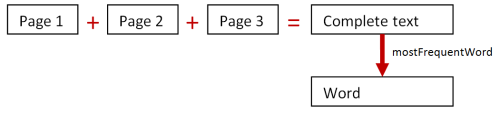The second approach will do `mostFrequentWord` separately on each page and then combine the results, like this: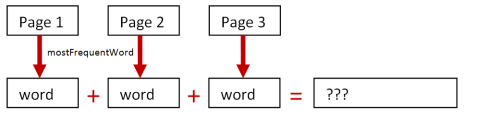Here’s the code:

``````module FrequentWordTest =

// code as above

document()
|> mostFrequentWord
|> printfn "Using add first, the most frequent word is %s"

document()
|> List.map mostFrequentWord
|> List.reduce (+)
|> printfn "Using map reduce, the most frequent word is %s"
``````

Can you see what happened? The first approach was correct. But the second approach gave a completely wrong answer!

``````Using add first, the most frequent word is world
Using map reduce, the most frequent word is hellogoodbyefoobar
``````

The second approach just concatenated the most frequent words from each page. The result is a new string that was not on any of the pages. A complete fail!

What went wrong?

Well, strings are a monoid under concatenation, so the mapping transformed a monoid (Text) to another monoid (string).

But the mapping did not preserve the “shape”. The most frequent word in a big chunk of text cannot be derived from the most frequent words in smaller chunks of text. In other words, it is not a proper monoid homomorphism.

### Definition of a monoid homomorphism

Let’s look at these two different examples again to understand what the distinction is between them.

In the word count example, we got the same final result whether we added the blocks and then did the word count, or whether we did the word counts and then added them together. Here’s a diagram: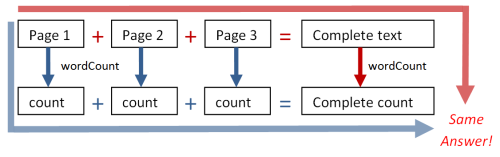But for the most frequent word example, we did not get the same answer from the two different approaches.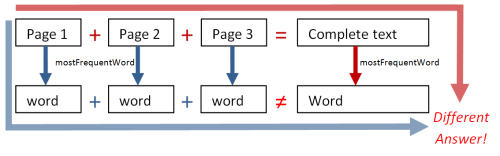In other words, for `wordCount`, we had

``````wordCount(page1) + wordCount(page2) EQUALS wordCount(page1 + page)
``````

But for `mostFrequentWord`, we had:

``````mostFrequentWord(page1) + mostFrequentWord(page2) NOT EQUAL TO mostFrequentWord(page1 + page)
``````

So this brings us to a slightly more precise definition of a monoid homomorphism:

``````Given a function that maps from one monoid to another (like 'wordCount' or 'mostFrequentWord')

Then to be a monoid homomorphism, the function must meet the requirement that:

function(chunk1) + function(chunk2) MUST EQUAL function(chunk1 + chunk2)
``````

Alas, then, `mostFrequentWord` is not a monoid homomorphism.

That means that if we want to calculate the `mostFrequentWord` on a large number of text files, we are sadly forced to add all the text together first, and we can’t benefit from a divide and conquer strategy.

… or can we? Is there a way to turn `mostFrequentWord` into a proper monoid homomorphism? Stay tuned!

## Next steps

So far, we have only dealt with things that are proper monoids. But what if the thing you want to work with is not a monoid? What then?

In the next post in this series, I’ll give you some tips on converting almost anything into a monoid.

We’ll also fix up the `mostFrequentWord` example so that it is a proper monoid homomorphism, and we’ll revisit the thorny problem of zeroes, with an elegant approach for creating them.

See you then!